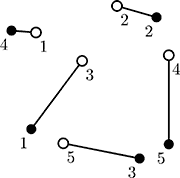#poj 3565 (弱校联萌十一大决战之厉兵秣马 A. Ants )

0
0
01. 云栖社区>
2. 博客>
3. 正文

## poj 3565 (弱校联萌十一大决战之厉兵秣马 A. Ants )

angel_imp 2015-10-05 13:06:00 浏览938

Ants
 Time Limit: 5000MS Memory Limit: 65536K Total Submissions: 5357 Accepted: 1660 Special Judge

Description

Young naturalist Bill studies ants in school. His ants feed on plant-louses that live on apple trees. Each ant colony needs its own apple tree to feed itself.

Bill has a map with coordinates of n ant colonies and n apple trees. He knows that ants travel from their colony to their feeding places and back using chemically tagged routes. The routes cannot intersect each other or ants will get confused and get to the wrong colony or tree, thus spurring a war between colonies.

Bill would like to connect each ant colony to a single apple tree so that all n routes are non-intersecting straight lines. In this problem such connection is always possible. Your task is to write a program that finds such connection.On this picture ant colonies are denoted by empty circles and apple trees are denoted by filled circles. One possible connection is denoted by lines.

Input

The first line of the input file contains a single integer number n (1 ≤ n ≤ 100) — the number of ant colonies and apple trees. It is followed by n lines describing n ant colonies, followed by n lines describing n apple trees. Each ant colony and apple tree is described by a pair of integer coordinates x and y (−10 000 ≤ xy ≤ 10 000) on a Cartesian plane. All ant colonies and apple trees occupy distinct points on a plane. No three points are on the same line.

Output

Write to the output file n lines with one integer number on each line. The number written on i-th line denotes the number (from 1 to n) of the apple tree that is connected to the i-th ant colony.

Sample Input

```5
-42 58
44 86
7 28
99 34
-13 -59
-47 -44
86 74
68 -75
-68 60
99 -60```

Sample Output

```4
2
1
5
3```

```#include <iostream>
#include <cstdio>
#include <cstring>
#include <cstdlib>
#include <cmath>
#include <vector>
#include <queue>
#include <algorithm>
#include <set>
using namespace std;

#define MM(a) memset(a,0,sizeof(a))

typedef long long LL;
typedef unsigned long long ULL;
const int maxn = 100000+5;
const int mod = 1000000007;
const double eps = 1e-7;

struct Point
{
double x, y;
}white, black;
///叉积
double xmul(Point p0, Point p1, Point p2)
{
return (p1.x-p0.x)*(p2.y-p0.y) - (p1.y-p0.y)*(p2.x-p0.x);
}
///判断条件
bool Judge(Point a, Point b, Point aa, Point bb)
{
if(max(a.x,b.x)>=min(aa.x,bb.x) && max(aa.x,bb.x)>=min(a.x,b.x)
&&max(a.y,b.y)>=min(aa.y,bb.y) && max(aa.y,bb.y)>=min(a.y,b.y))
if(xmul(a,b,aa)*xmul(a,b,bb) < -eps)
if(xmul(aa,bb,a)*xmul(aa,bb,b) < -eps)
return true;
return false;
}
int n, match;
void Init()
{
for(int i=1; i<=n; i++)
match[i]=i;
}
int main()
{
while(~scanf("%d",&n))
{
Init();
for(int i=1; i<=n; i++)
scanf("%lf%lf",&white[i].x,&white[i].y);
for(int i=1; i<=n; i++)
scanf("%lf%lf",&black[i].x,&black[i].y);
while(1)
{
bool ok=true;
///冒泡
for(int i=1; i<=n; i++)
for(int j=1; j<=n; j++)
{
if(i==j)
continue;
if(Judge(white[i],black[match[i]],white[j],black[match[j]]))
{
swap(match[i],match[j]);
ok=false;
}
}
if(ok)
break;
}
for(int i=1; i<=n; i++)
printf("%d\n",match[i]);
}
return 0;
}
```

angel_imp
+ 关注